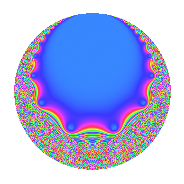# Properties

 Label 20.12.a.bLevel 20 Weight 12 Character orbit 20.a Self dual Yes Analytic conductor 15.367 Analytic rank 0 Dimension 2 CM No Inner twists 1

# Related objects

## Newspace parameters

 Level: $$N$$ = $$20 = 2^{2} \cdot 5$$ Weight: $$k$$ = $$12$$ Character orbit: $$[\chi]$$ = 20.a (trivial)

## Newform invariants

 Self dual: Yes Analytic conductor: $$15.3668636112$$ Analytic rank: $$0$$ Dimension: $$2$$ Coefficient field: $$\Q(\sqrt{46729})$$ Coefficient ring: $$\Z[a_1, a_2, a_3]$$ Coefficient ring index: $$2^{2}$$ Fricke sign: $$1$$ Sato-Tate group: $\mathrm{SU}(2)$

## $q$-expansion

Coefficients of the $$q$$-expansion are expressed in terms of $$\beta = 2\sqrt{46729}$$. We also show the integral $$q$$-expansion of the trace form.

 $$f(q)$$ $$=$$ $$q + ( 110 - \beta ) q^{3} + 3125 q^{5} + ( 1670 - 111 \beta ) q^{7} + ( 21869 - 220 \beta ) q^{9} +O(q^{10})$$ $$q + ( 110 - \beta ) q^{3} + 3125 q^{5} + ( 1670 - 111 \beta ) q^{7} + ( 21869 - 220 \beta ) q^{9} + ( 149160 + 390 \beta ) q^{11} + ( 604910 + 3156 \beta ) q^{13} + ( 343750 - 3125 \beta ) q^{15} + ( 4528170 + 5028 \beta ) q^{17} + ( 9719684 - 15180 \beta ) q^{19} + ( 20931376 - 13880 \beta ) q^{21} + ( 27963210 - 6537 \beta ) q^{23} + 9765625 q^{25} + ( 24040940 + 131078 \beta ) q^{27} + ( 20920854 + 336840 \beta ) q^{29} + ( 31115396 - 593250 \beta ) q^{31} + ( -56489640 - 106260 \beta ) q^{33} + ( 5218750 - 346875 \beta ) q^{35} + ( -364617970 + 731304 \beta ) q^{37} + ( -523366796 - 257750 \beta ) q^{39} + ( -304209534 - 629460 \beta ) q^{41} + ( -564720370 + 1748715 \beta ) q^{43} + ( 68340625 - 687500 \beta ) q^{45} + ( -826536450 + 3182673 \beta ) q^{47} + ( 328454193 - 370740 \beta ) q^{49} + ( -441714948 - 3975090 \beta ) q^{51} + ( 2862443670 - 3916068 \beta ) q^{53} + ( 466125000 + 1218750 \beta ) q^{55} + ( 3906550120 - 11389484 \beta ) q^{57} + ( 1878216948 + 8296440 \beta ) q^{59} + ( 2461851782 + 20736720 \beta ) q^{61} + ( 4601009950 - 2794859 \beta ) q^{63} + ( 1890343750 + 9862500 \beta ) q^{65} + ( -2921622070 - 6916671 \beta ) q^{67} + ( 4297822992 - 28682280 \beta ) q^{69} + ( -21676081332 + 8865270 \beta ) q^{71} + ( 16823549810 + 11326356 \beta ) q^{73} + ( 1074218750 - 9765625 \beta ) q^{75} + ( -7842496440 - 15905460 \beta ) q^{77} + ( -27399712648 - 40981860 \beta ) q^{79} + ( -25730099791 + 29349980 \beta ) q^{81} + ( -4651516050 + 14154423 \beta ) q^{83} + ( 14150531250 + 15712500 \beta ) q^{85} + ( -60659491500 + 16131546 \beta ) q^{87} + ( 1844484186 + 168050520 \beta ) q^{89} + ( -64469465756 - 61874490 \beta ) q^{91} + ( 114310610560 - 96372896 \beta ) q^{93} + ( 30374012500 - 47437500 \beta ) q^{95} + ( 32946078890 + 111643644 \beta ) q^{97} + ( -12775412760 - 24286290 \beta ) q^{99} +O(q^{100})$$ $$\operatorname{Tr}(f)(q)$$ $$=$$ $$2q + 220q^{3} + 6250q^{5} + 3340q^{7} + 43738q^{9} + O(q^{10})$$ $$2q + 220q^{3} + 6250q^{5} + 3340q^{7} + 43738q^{9} + 298320q^{11} + 1209820q^{13} + 687500q^{15} + 9056340q^{17} + 19439368q^{19} + 41862752q^{21} + 55926420q^{23} + 19531250q^{25} + 48081880q^{27} + 41841708q^{29} + 62230792q^{31} - 112979280q^{33} + 10437500q^{35} - 729235940q^{37} - 1046733592q^{39} - 608419068q^{41} - 1129440740q^{43} + 136681250q^{45} - 1653072900q^{47} + 656908386q^{49} - 883429896q^{51} + 5724887340q^{53} + 932250000q^{55} + 7813100240q^{57} + 3756433896q^{59} + 4923703564q^{61} + 9202019900q^{63} + 3780687500q^{65} - 5843244140q^{67} + 8595645984q^{69} - 43352162664q^{71} + 33647099620q^{73} + 2148437500q^{75} - 15684992880q^{77} - 54799425296q^{79} - 51460199582q^{81} - 9303032100q^{83} + 28301062500q^{85} - 121318983000q^{87} + 3688968372q^{89} - 128938931512q^{91} + 228621221120q^{93} + 60748025000q^{95} + 65892157780q^{97} - 25550825520q^{99} + O(q^{100})$$

## Embeddings

For each embedding $$\iota_m$$ of the coefficient field, the values $$\iota_m(a_n)$$ are shown below.

For more information on an embedded modular form you can click on its label.

Label $$\iota_m(\nu)$$ $$a_{2}$$ $$a_{3}$$ $$a_{4}$$ $$a_{5}$$ $$a_{6}$$ $$a_{7}$$ $$a_{8}$$ $$a_{9}$$ $$a_{10}$$
1.1
 108.584 −107.584
0 −322.338 0 3125.00 0 −46319.5 0 −73245.3 0
1.2 0 542.338 0 3125.00 0 49659.5 0 116983. 0
 $$n$$: e.g. 2-40 or 990-1000 Significant digits: Format: Complex embeddings Normalized embeddings Satake parameters Satake angles

## Inner twists

This newform does not admit any (nontrivial) inner twists.

## Atkin-Lehner signs

$$p$$ Sign
$$2$$ $$-1$$
$$5$$ $$-1$$

## Hecke kernels

This newform can be constructed as the kernel of the linear operator $$T_{3}^{2} - 220 T_{3} - 174816$$ acting on $$S_{12}^{\mathrm{new}}(\Gamma_0(20))$$.